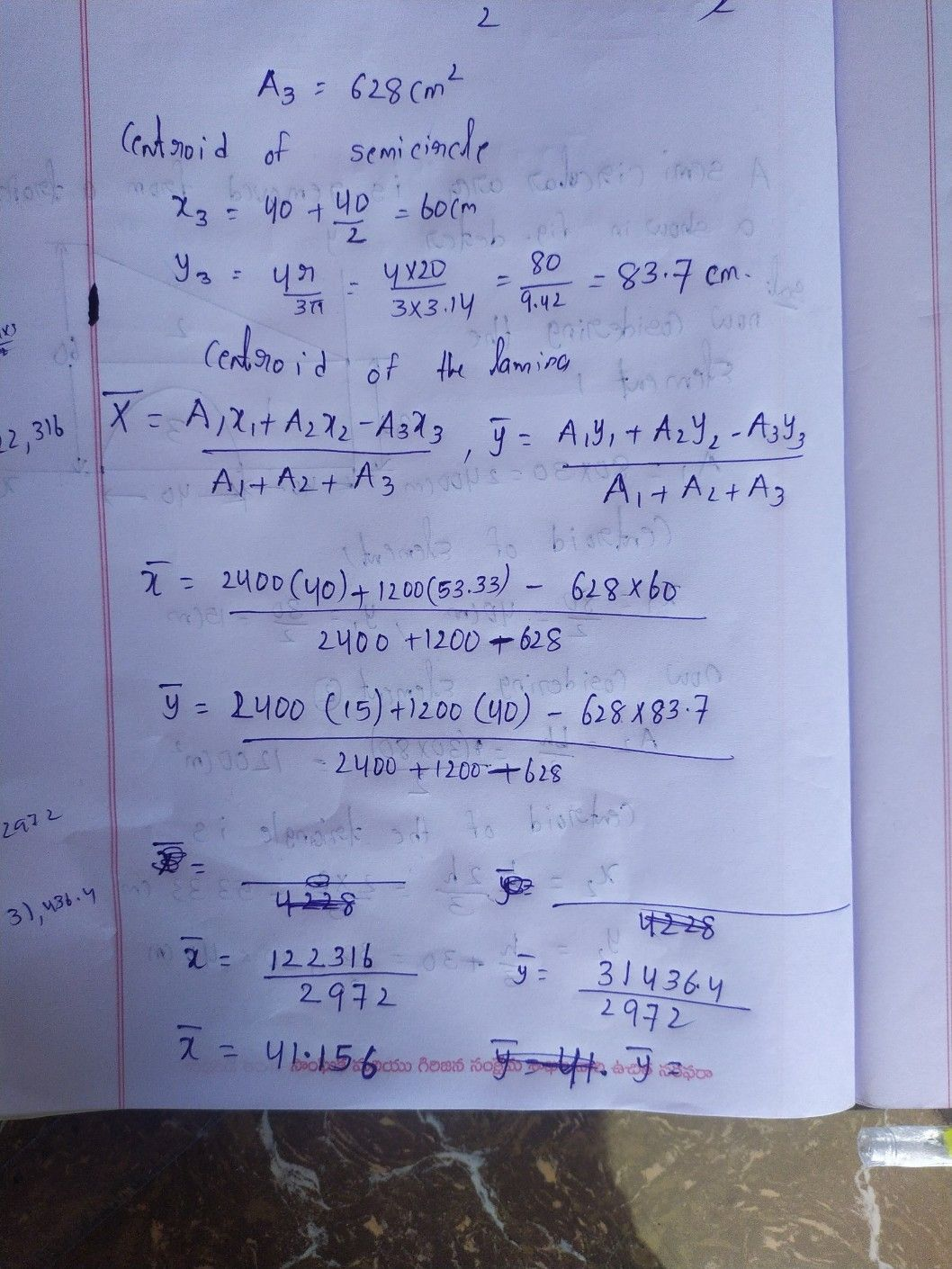Symbol
Problem$1f$ $l\left(f\left(t\right)=F\left(5\right),$ $,$ $,$ then $m_{z}→\infty ^{f\left(t\right)}$ $=lim _{s→0}$ $sF\left(5\right)$ is called
Other
Search count: 107
SolutionQanda teacher - JagadeshC